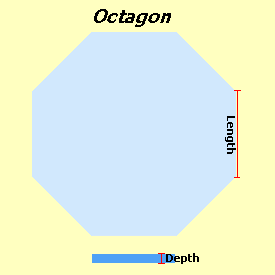Spike's Calculators

# Concrete Calculator for an Octagon Shaped SlabCalculate the amount of concrete you need for a slab in the shape of an octagon using metres and millimetres.

In a regular Octagon, there are eight straight, equal-length sides and eight inside corners that total 135°. To calculate the volume of an Octagon shaped slab, you need to provide the length of one side in metres and the required depth of the slab in millimetres.

### Octagon Shaped Volume Calculator - Metric

Side Length:   m
Depth:   mm
Area:   m ²
Perimeter:   lin m

#### Amount of concrete needed:

Cubic Meters:   m ³
Cubic Yards:   yd ³

#### Calculation

1. enter the length of a side in metres
2. the slab thickness (depth) in millimetres

#### Results

1. the area of the slab in square metres
2. the perimeter of the slab in lineal metres for forming material
3. the amount of concrete needed in cubic metres
4. in cubic yards

### When Ordering Concrete

This concrete calculator will help you in estimating the amount of concrete needed for your project. The amount given as needed, does not include any waste. It is recommended, depending on the job you are doing, to add anywhere from an extra 4% to 10% to your concrete order to make sure you have enough concrete to finish the job. Ask when ordering the concrete. They will be able to give you a good idea of what is needed.